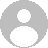# เผยแพร่ผลงานทางวิชาการฉบับแปลเป็นภาษาอังกฤษ

สูรย์ สุขจิต
ติดตาม ผู้ติดตาม
Publishing scholarly works.

Publishing scholarly works.

Title:    Results of teaching math using math games with instruction set as
Lesson plans on the normal factor of hundreds. For students.
Grade 6, Ban Kut  Kho Kan Khok  Samran school.

Name of study: Mr. Soon Sukjit

Abstract.
Were taught mathematics using the set of math games to teach lesson plans based on the normal factor of hundreds. For students in Grade 6, Ban Kut  Kho Kan Khok  Samran school. Aims are: (1) to determine the efficiency of teaching mathematics using set of math games. To teach the lesson plans on the normal factor of hundreds. For students in Grade 6, Ban Kut  Kho Kan Khok  Samran school  basis 75/75 (2) to determine the effectiveness index of teaching mathematics using set of math games. To teach the lesson plans on the normal factor of hundreds. For students grade 6, Ban Kut  Kho Kan Khok  Samran school  and (3) to compare academic achievement in mathematics and mathematics teaching by using the set of math games. To teach the lesson plans on regular. Factor of hundreds. For students grade 6, Ban Kut  Kho Kan Khok  Samran school. The population in this study. Students in Grade 6, Ban Kut  Kho Kan Khok  Samran school. The network quality of a Ming - Khok Samran District Loeng Nok Tha Yasothon Provincial Office of Education Area Yasothon 2 in year 2551, 3 classroom students were 89 people using a sample of 44 people by drawing lots in pairs (. Match-pair Technique) is divided into an experimental group and the control groups of 22 persons with a step in the study were (1) test before learning (Pre-Test) using the test achievement of the study made before will be taught by a series of math games on hundreds of items and 30 items (2) experimental group 1 (experimental group) to carry out a series of teaching math games on hundreds of factors. And group 2 (control group) and the instructional lesson plans created on the normal factor of hundreds. Time was 21 hours, was carried out in the first semester of the academic year 2551 during May-August 2551 (3) after learning test (Post-Test) at the end of the game with a set of teaching mathematics on the cardinal factor. With a test version the same achievement tests prior to and (4), data from achievement tests. Statistical analysis to determine the next

The results are summarized as follows.

1. Students grade 6, the experimental group learned with a set of math games and 19 series' average score was 84.31 out of 95 points per cent 88.75 The students grade 6, a control group learning plans. Learn the conventional 19 plan mean score was 62.90 out of 95 points per cent 66.22, and when analyzed for efficiency of teaching mathematics with a set of math games to teach the lesson plans were found that the effectiveness of learning. set of math games on hundreds of factors. For students grade 6, the experimental group. 88.75/91.21 effective 75/75, which is higher than the standard set.
2. Index to study the effectiveness of the experimental group with a set of math games on hundreds of factors. For students grade 6, is equal to 0.8632 indicates that student progress in learning mathematics on hundreds of factors. 86.32 per cent.
3. Achievement scores after learning of the experimental group and control group learning with a set of math games and learning with lesson plans for regular For students grade 6, Ban Kut  Kho Kan Khok  Samran school. The difference statistically significant at the .01 level.

#### ความเห็น (4)Nipon Thongkote
IP: xxx.26.205.35
เขียนเมื่อ

excellanceThank you very much for visiting me online.waranee phumee
IP: xxx.26.96.3
เขียนเมื่อ

Good  Ideal